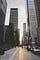# AI Sidewalk #3: Delving Into Binary SVMs(Part 2)Beginning of a new journey. Credit to www.pexels.com/@tony-367405

# Last Time

Here’s a link to the part 1 article: https://medium.com/@ssp247/ai-sidewalk-2-delving-into-binary-svms-part-1-d304907dd520

# Lagrangian Duality Explored

Last time we saw the following optimization:

and its corresponding dual problem:

This section first goes into the details of general Lagrangian and then the . details of how we go about converting the first minimization into the second maximization.

## The Lagrangian

In general the Lagrangian is just a way of solving optimization problems. Given the general optimization

Its corresponding Lagrangian would be

## Duality

In general suppose we are given an optimization in the form

If we switch the maximization and minimization operations we end up with

Here g(y) is the dual function of f(x,y).

## Duality Gap and Strong Duality

Theoretically, if the solution to the original optimization from above is s1 and the solution to its dual is s2, we define the notion of optimal duality gap as being the difference between these two solutions (s1-s2). Furthermore, strong duality is when the solutions to the primal and dual are equal (s1=s2).

## KKT (Karush-Kuhn-Tucker) Conditions

Given that strong duality exists, we can use the Karush-Kuhn-Tucker conditions in order to solve for the duality we derive.

• 1st KKT Condition (Stationarity): Given that (x*, \lambda*) is the optimal solution for our Lagrangian and that strong duality holds, the first KKT condition would hold true:
• 2nd KKT Condition (Complementary Slackness): Since we are given that g_{i} ≤ 0 and lambda_{i} ≥ 0 for all i via primal feasibility, and taking into account the first condition from above we derive the following conditions:

# Calculating Duality for Soft-Margin SVMs

First we can start off by converting all of the conditions into the general form we have seen above:

Now we plug in these derived inequality constraints into the Lagrangian formula as follows:

The base-level optimization for the Lagrangian is:

Just to make the notation clear, here epsilon, alpha and beta are n-element vectors of each of the individual alpha, beta and epsilon values:

If we switch the maximization and minimization, we can calculate the dual function of the Lagrangian:

We can now simply minimize the function g by carrying out the usual optimization steps. The first thing to do is to calculate the proper derivatives and set them equal to zero:

Notice that solving for w in the first expression above is rather tedious. Substituting the L2-norm of w with half of the square of the L2-norm of w in the original optimization is often used as a work-around for calculating a cleaner solution without changing the nature of the problem (we are still focused minimizing w).

After this tweak, the following is the optimal value we get for w:

Instead of providing us any optimal values, the other derivatives provide us a set of constraints for that have to be met for the second (outer) optimization:

Do you notice something about what we have derived above, and the dual optimization at the very top of the post? If you see some similarity, you aren’t mistaken! We have this outer optimization problem after plugging in the constraints from above:

This objective is the exact same as the objective from above! Finally, let’s try reasoning out the constraints. Well, the first constraint has already been derived by us earlier:

and for the second constraint we just need to manipulate an existing condition and utilize the facts that C needs to be greater than or equal to 0 (where C=0 leads to a Hard-Margin SVM!) and the betas also need to be greater than or equal to 0:

## Extension

What is the Stationarity KKT Condition for the above case? What about the Complementary Slackness KKT Condition?

## Big Picture

The whole point of going through the calculations above we to go through the math behind transforming an optimization involving many variables into one involving only a single vector. This result is much easier to optimize than what we started off with!

# Brief Intro to Kernels

There will be a separate post in the future covering the concept of kernels in more depth, but essentially kernels are just a means of helping us “transform” the given data to be more linearly separable.

Specifically in the context of SVMs, there are several different types of kernels that are commonly utilized:

# Next Time

Next time we will code up a binary SVM from scratch, train and test it on the University of Wisconsin Breast Cancer Dataset, and perform an analysis of the results!

Written by